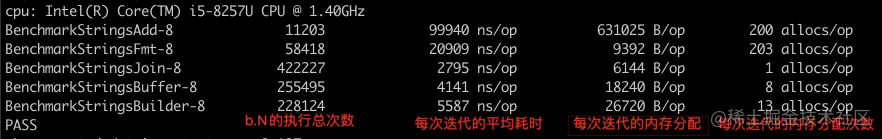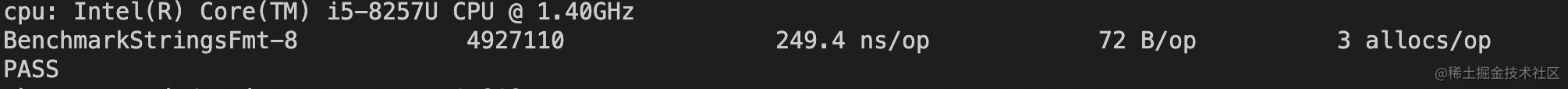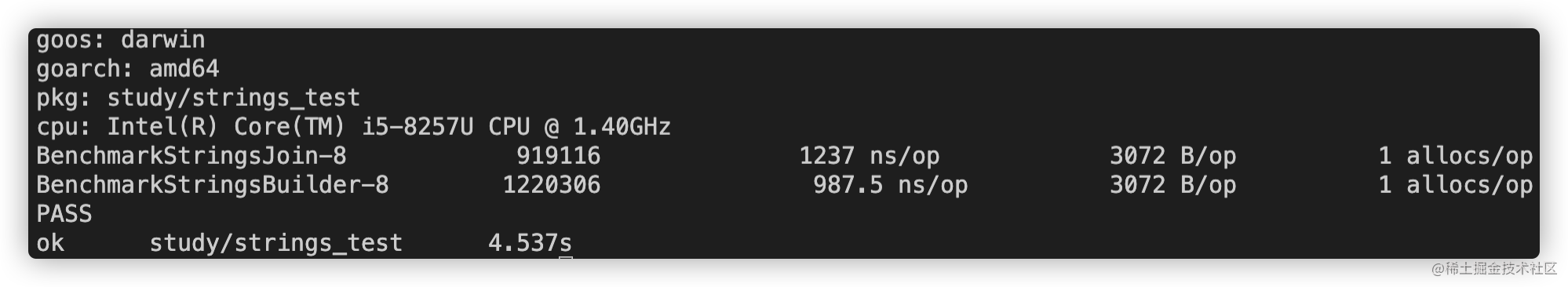# 0 准备工作

benchmark是Go自带的测试利器，使用benchmark我们可以方便快捷的测试一个函数方法在串行和并行环境下的表现，指定一个时间（默认测试1秒），看被测方法在达到这个时间上限所能执行的次数和内存分配情况。

benchmark的常用API有如下几种：

``````// 开始计时
b.StartTimer()
// 停止计时
b.StopTimer()
// 重置计时
b.ResetTimer()
b.Run(name string, f func(b *B))
b.RunParallel(body func(*PB))
b.ReportAllocs()
b.SetParallelism(p int)
b.SetBytes(n int64)
testing.Benchmark(f func(b *B)) BenchmarkResult

``````b.StartTimer()   // 开始计时
b.StopTimer()    // 停止计时
b.ResetTimer()   // 重置计时

# 1 构建测试用例

``````var StrData = []string{"Go语言高效拼接字符串"}

``````func init() {
for i := 0; i < 200; i++ {
StrData = append(StrData, "Go语言高效拼接字符串")
}
}

## 1.1 “+”直接拼接

``````func StringsAdd() string {
var s string
for _, v := range StrData {
s += v
}
return s
}
// 测试方法
b.ResetTimer()
for i := 0; i < b.N; i++ {
}
b.StopTimer()
}

## 1.2 使用fmt包进行组装

``````func StringsFmt() string {
var s string = fmt.Sprint(StrData)
return s
}

// 测试方法
func BenchmarkStringsFmt(b *testing.B) {
b.ResetTimer()
for i := 0; i < b.N; i++ {
StringsFmt()
}
b.StopTimer()
}

## 1.3 使用strings包的join方法

``````func StringsJoin() string {
var s string = strings.Join(StrData, "")
return s
}

// 测试方法
func BenchmarkStringsJoin(b *testing.B) {
b.ResetTimer()
for i := 0; i < b.N; i++ {
StringsJoin()
}
b.StopTimer()
}

## 1.4 使用bytes.Buffer拼接

``````func StringsBuffer() string {
var s bytes.Buffer
for _, v := range StrData {
s.WriteString(v)
}
return s.String()
}
// 测试方法
func BenchmarkStringsBuffer(b *testing.B) {
b.ResetTimer()
for i := 0; i < b.N; i++ {
StringsBuffer()
}
b.StopTimer()
}

## 1.5 使用strings.Builder拼接

``````func StringsBuilder() string {
var b strings.Builder
for _, v := range StrData {
b.WriteString(v)
}
return b.String()
}
// 测试方法
func BenchmarkStringsBuilder(b *testing.B) {
b.ResetTimer()
for i := 0; i < b.N; i++ {
StringsBuilder()
}
b.StopTimer()
}

# 2 测试结果及分析

## 2.1 使用benchmark运行测试，查看结果• 我们直接使用“+”号拼接是耗时最多，内存消耗最大的操作，b.N周期内的每次迭代，都会进行内存分配；
• 使用Strings.Join方法进行拼接的执行的次数最多，代表耗时最小，而内存的分配次数最少，每次迭代分配的内存也是最少的；
• 使用Strings.Builder类型进行拼接，每次迭代都额外分配了13次内存，性能并没有明显的优势，可是Go从1.10开始新增了这个类型，并开始逐步使用呢？

## 2.3 fmt包进行拼接的结果分析``````# 使用benchmark采集3秒的数据，并生成文件
go test -bench=. -benchmem  -benchtime=3s -memprofile=mem_profile.out
# 查看pprof文件，指定http方式查看
go tool pprof -http="127.0.0.1:8080" mem_profile.out

## 2.4 使用strings.Join方法进行拼接的结果分析

``````// Join concatenates the elements of its first argument to create a single string. The separator
// string sep is placed between elements in the resulting string.
func Join(elems []string, sep string) string {
switch len(elems) {
case 0:
return ""
case 1:
return elems
}
n := len(sep) * (len(elems) - 1)
for i := 0; i < len(elems); i++ {
n += len(elems[i])
}

var b Builder
b.Grow(n)
b.WriteString(elems)
for _, s := range elems[1:] {
b.WriteString(sep)
b.WriteString(s)
}
return b.String()
}

## 2.5 使用bytes.Buffer方法进行拼接的结果分析

``````// A Buffer is a variable-sized buffer of bytes with Read and Write methods.
// The zero value for Buffer is an empty buffer ready to use.
type Buffer struct {
buf      []byte // contents are the bytes buf[off : len(buf)]
off      int    // read at &buf[off], write at &buf[len(buf)]
}

## 2.6 使用strings.Builder方法进行拼接的结果分析

``````// A Builder is used to efficiently build a string using Write methods.
// It minimizes memory copying. The zero value is ready to use.
// Do not copy a non-zero Builder.
type Builder struct {
buf  []byte
}

func (b *Builder) copyCheck() {
// This hack works around a failing of Go's escape analysis
// that was causing b to escape and be heap allocated.
// See issue 23382.
// TODO: once issue 7921 is fixed, this should be reverted to
} else if b.addr != b {
panic("strings: illegal use of non-zero Builder copied by value")
}
}

// String returns the accumulated string.
func (b *Builder) String() string {
return *(*string)(unsafe.Pointer(&b.buf))
}

// WriteString appends the contents of s to b's buffer.
// It returns the length of s and a nil error.
func (b *Builder) WriteString(s string) (int, error) {
b.copyCheck()
b.buf = append(b.buf, s...)
return len(s), nil
}

// grow copies the buffer to a new, larger buffer so that there are at least n
// bytes of capacity beyond len(b.buf).
func (b *Builder) grow(n int) {
buf := make([]byte, len(b.buf), 2*cap(b.buf)+n)
copy(buf, b.buf)
b.buf = buf
}

# 3 strings.Builder和strings.Join的比较

strings.Join的关键代码：

``````func Join(elems []string, sep string) string {
switch len(elems) {
case 0:
return ""
case 1:
return elems
}
n := len(sep) * (len(elems) - 1)
for i := 0; i < len(elems); i++ {
n += len(elems[i])
}

var b Builder
b.Grow(n)
b.WriteString(elems)
for _, s := range elems[1:] {
b.WriteString(sep)
b.WriteString(s)
}
return b.String()
}

``````func StringsBuilder() string {

var b strings.Builder
for _, v := range StrData {
b.WriteString(v)
}

return b.String()
}

``````func StringsBuilder() string {

n := len("") * (len(StrData) - 1)
for i := 0; i < len(StrData); i++ {
n += len(StrData[i])
}

var b strings.Builder
b.Grow(n)
b.WriteString(StrData)
for _, s := range StrData[1:] {
b.WriteString("")
b.WriteString(s)
}
return b.String()
}``````func StringsBuilder(strData []string,sep string) string {

n := len(sep) * (len(strData) - 1)
for i := 0; i < len(strData); i++ {
n += len(strData[i])
}

var b strings.Builder
b.Grow(n)
b.WriteString(strData)
for _, s := range strData[1:] {
b.WriteString(sep)
b.WriteString(s)
}
return b.String()
}

# 5 总结

strings.Builder ≈ strings.Join > strings.Buffer > "+" > fmt